Courses

# Eigen Function And Eigen Value Equation - Atomic Structure Chemistry Notes | EduRev

## Chemistry : Eigen Function And Eigen Value Equation - Atomic Structure Chemistry Notes | EduRev

The document Eigen Function And Eigen Value Equation - Atomic Structure Chemistry Notes | EduRev is a part of the Chemistry Course Physical Chemistry.
All you need of Chemistry at this link: Chemistry

EIGEN FUNCTION AND EIGEN VALUE EQUATION

Eigen value equations are those equations in which on the operating of a function by an operator, we get function back only multiplied by a constant value. The function is called eigen function and the constant value is
called eigen value.

Show that eax is the eigen function of operator.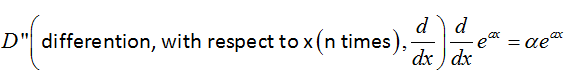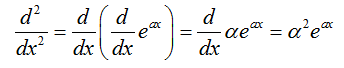Similarly,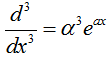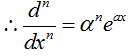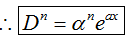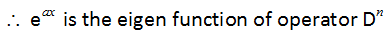Concept of Wave function:

Quantum mechanics acknowledges the wave-particle duality of matter and the existence of quantization by supporting that, rather than travelling along a definite path, a particle is distributed through apace like a wave. The mathematical representation of the wave that in quantum mechanics replaces the classical concept of trajectory is called a wave function,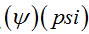.The Schrodinger equation is a second-order differential equation used to calculate the wave function of a system.

The Schrodinger equation:
For one = dimensional system,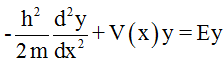Where V(x) is the potential energy of the particle and E is its total energy. For three-dimensional system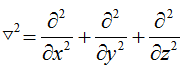In systems with spherical symmetry three equivalent forms are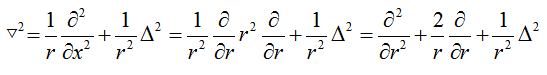Where,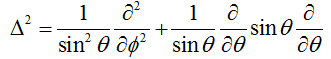In the general case the Schrodinger equation is written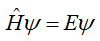Where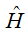is the Hamiltonian operator for the system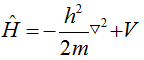For the evolution of a system with time, it is necessary to solve the time-dependent equation: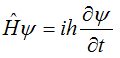A central principle of quantum mechanics is that wave function contains all the dynamical information about the system it is describes. Here we concentrate on the information it carries about the location of the particle.

The interpretation of the wave function in terms of the location of the particle is based on a suggestion made by Max Born. He made use of an analogy with the wave theory of light, in which the square of the amplitude of an electromagnetic wave in a region is interrupt as its intensity and therefore (in quantum terms) as a measure of the probably of finding a photon present in the region. The Born interpretation of the wave function focuses on the square of the wave function.

If the wave function of a particle has the value ψ(x) at some point x, then the probably of finding the particle between x and x+ dx is proportional to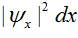Thus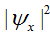is the probability density, and to obtain the probability it must be multiplied by the length of the infinitesimal region dx. The wave function ψitself is called the probability amplitude.

for a particle free to move in three dimensions (for example, an electron near a nucleus in an atom), the wave function depends on the point x with coordinates x, y and z, and the interpretation of ψ(r) is as follows. If the wave function of a particle; has the value ψ at some point 'r' , then  the probability of finding the particle in an infinitesimal volume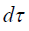=dxdydz at that point is proportional to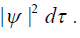The square of a wave function is a probability density, and (in three dimensions) has the dimensions of 1/length3. It becomes a (unit less) probability when multiplied by a volume. In general, we have to take the account the variation of the amplitude of the wave function over the volume of interest, but here we are supposing that the volume is so small that the variation of ψ in the region can be ignored.

Mathematical feature of the Schrodinger equation is that, if ψ is a solution, then si is Nψ, where N is any constant. This freedom to vary the wave function by a constant factor means that it is always possible to find normalization constant, N, such that the proportionality of the born interpretation becomes an equality.

We find the normalization constant by noting that, for a normalized wave function Nψ the probability that a particle is in the region dx is equal to (Nψ)(Nψ)dx (we are taking N to be real). Furthermore, the sum over all space of these individual probabilities must be1.

The integration is over all the space accessible to the particle. For systems With spherical symmetry it is best to work in spherical polar coordinates r, θ and φ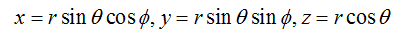R, the radius, ranges from 0 to ∞

θ , the colatitudes, ranges from 0 to π

φ , the azimuthal, ranges from 0 to 2π

Standard manipulation then yield.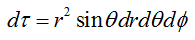Conditions of acceptable wave function:

• Continuous

• Have a continuous slope

• Be a single-valued

• Should be finite.

A particle may possess only certain energies for otherwise its wave function would be physically unacceptable.
That is, as a consequence of the restriction on its wave function, the energy is quantized.
The wave function must satisfy stringent conditions for it to be acceptable.

1. Unacceptable because it is not continuous

2. Unacceptable because its slope is discontinuous

3. Unacceptable because it is not single-valued

4. Unacceptable because it is infinite over a finite region

We have claimed that a wave function contains all the information it is possible to obtain about the dynamical properties of the particle (for example, its location and momentum). We have seen that the Born interpretation tells us much as we can know about location, but how do we find any additional dynamical information.

PROBABILITY DENSITY

Probability of finding a particle within a limit from lower limit to upper limit may be calculated as: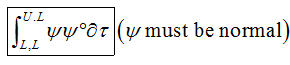Q. Calculated the probability that a particle in a 1-D box of length a is found to be between 0 and a/2
Sol. Normalized wave function for 1-D box=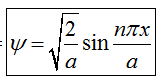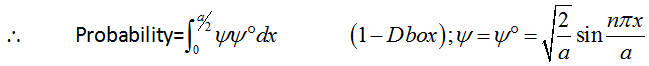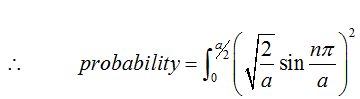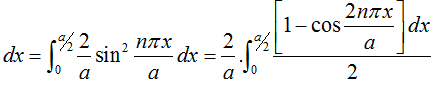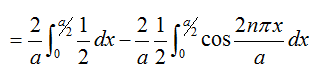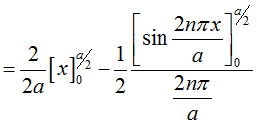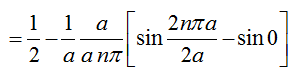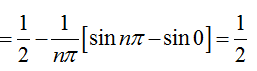NORMALISATION

Normalization means to bring a function in normal state. If a wave function satisfies this equation. then it is said to normalized.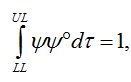Note: UL= Upper limit
LL=Lower limit.

In quantum mechanics, ψ  is obtained by solving wave equation is known a normalized wave function.
(Very often or generally)

We know that it is possible to multiply ψ by a constant A to give a new wave function, Aψ, which is also a solution of wave equation, now the problem id to choose the proper value of A, such that the new wave function is a normalized function in it is a normalized wave function, it must meet the requirement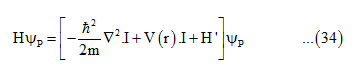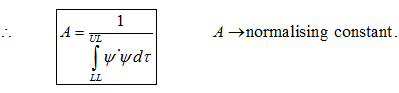Q. A wave function given by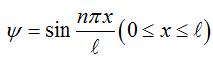is it normalized. If not normalize it.

Sol.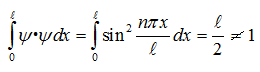So, the function is not normalized. To normalize this wave function, a constant A is taken with the functionand now function becomes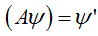So, according to the condition of normalization,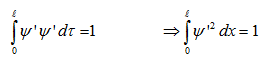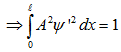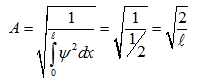ORTHOGONALITY, KRONECKER DELTA AND ORTHONORMAL SET:

If two wave function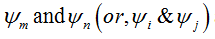a such that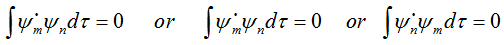Then ψand  ψn said to be orthogonal.

If m=n; in this condition. Then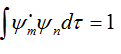normalised and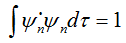normalised

A set of functions that are both normalized and orthogonal to each other is called a orthogonal set.

We can express the condition such that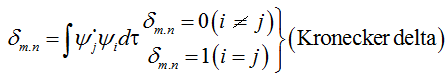The wave function of particle in 1-D box is classical example of orthonormal set.

Offer running on EduRev: Apply code STAYHOME200 to get INR 200 off on our premium plan EduRev Infinity!

## Physical Chemistry

66 videos|94 docs|32 tests

,

,

,

,

,

,

,

,

,

,

,

,

,

,

,

,

,

,

,

,

,

;